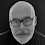## Wednesday, September 26, 2012

### How To... Draw a Basic Control Chart in Excel

Control Charts are considered to be one of the Seven Basic Tools of Quality. They are helpful in Quality Control Management to show if a process is in or out of control. The principle to drawing a Control Chart is fairly straight-forward, but it is not so easy to do in Excel because you need to add lines for Upper Control Limits (UCL), Lower Control Limits (LCL) and a Central Line (CL).

The video below shows a way to work around this by plotting four sets of data. The first is that actual data representing the quality measurements, the other three are "cheats". For each data point representing the measurements, plot the Mean, Standard Deviation + 3, and Standard Deviation - 3, in other words the same value over and over. This gives us straight lines as shown in the video. Check it out and see what you think:

1.Thank you very much. you made my life easier

2.Hi,
The type chart that you built in your video is it XBar S chart??
I am studying control charts, and I have found 3 Control Charts that I would
like to see in Excel.
The XBar R, XmR, and XBar S charts and the main purpose of each chart.
Are you planning to create more training videos?

Thanks

3.For this type of experiment, we cannot use the standard deviation function in excel. Since the underlying experiment is pass or fail, we have so-called Bernoulli trials, and we expect the distribution to be the binomial rather than the normal. so we should be plotting fraction defective, not number of defectives, and we should be using the formula for the standard deviation of a binomial distributed random variable.
Often in p-charts we use 2 standard deviations for the UCL and LCL computation. That is about 10% probability that the measurement is outside the lines.

1.Hi Anon,

I have had quite a bit of feedback about this video on my YouTube Channel and investigating the best calculation of Std Dev to use. Some use Excel to calculate StdDev, some use the square root of mean for it, and there are other ways too.

4.